WEBINAR
Brushless DC Permanent Magnet Motors: Design, Simulation, and Performance Evaluation
Thursday, October 19, 2023
Time
SESSION 1
SESSION 2
CEST (GMT +2)
03:00 PM
08:00 PM
EDT (GMT -4)
09:00 AM
02:00 PM
HOME / Applications / Modeling and simulation of Switched Reluctance Motor for electric vehicle inside SOLIDWORKS using EMWorks products

# Modeling and simulation of Switched Reluctance Motor for electric vehicle inside SOLIDWORKS using EMWorks products

## Introduction

A switched reluctance motor (SRM) is a rotating electric machine where both stator and rotor have salient poles (Figure 1). The stator winding comprises a set of coils, each of which is wound on one pole. The rotor is created from lamination in order to minimize the eddy current losses. The motor is excited by a sequence of current pulses applied at each phase, forcing the motor to rotate. The current pulses need to be applied to the respective phase at the exact rotor position relative to the excited phase.

FEM and CAD modeling of SRM are important because they save effort, time and money. The modeling and simulation start by a CAD design of an electrical machine. Later, the CAD geometry will be combined with numerical simulation using MotorWizard. The Finite Element Method (FEM), used in MotorWizard, has shown its reliability when dealing with electromagnetic design . In this article two-dimensional FEM is used to analyze the proposed motor. The 2D solution approximation is considered because of its capability to give fast and very close results compared to a real motor case. This solution helps to accelerate the modeling and design process. Magnetic flux density and static torque will be studied and computed versus rotor angle. The rotor is moving from unaligned position to the aligned position. These results will help to identify the peak values of both magnetic flux and static torque as well as their positions. The static torque results obtained by MotorWizard will be compared to the measurement data published in ref .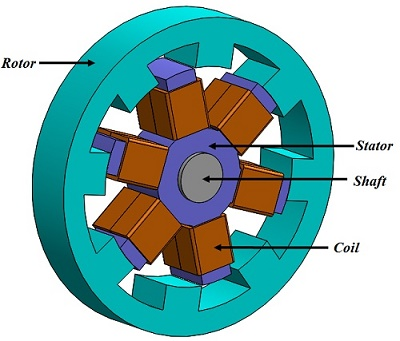Figure 1 - Switched reluctance motor

## EMWorks 's electric Motors design and simulation package

EMWorks offers MotorWizard: a full package seamlessly integrated inside SOLIDWORKS to create and simulate electrical motors.

## Geometry generation

### Step 1: Build the SRM

The first step in studying SRM is creating the 2D model. The main properties of the motor are listed in table 1 ref . After choosing the stator position of the motor (inner or outer position), different parameters have been specified for the motor as follows:

1. Define the general sizing setting of the machine: In these fields, the rotor and the stator number of poles and the phase number are specified. The poles number should be an even and conform with the phase number. This is automatically verified by MotorWizard.

2. Define the stator properties: it includes the outer and inner diameter of the stator, yoke thickness, stator arc angle and the length of stator in case of 3D model. The windings geometrical properties (insulation thickness, end adjustment for 3D models, etc) are also entered in this section.

3. Define the rotor properties: it contains the outer and inner diameter of the rotor, yoke thickness and stator arc angle and the length of rotor in case of 3D model.

4. Validate and generate the model: at this step, user can verify the consistency of his model before the creation.

Table 1 - SRM main properties
 Stator poles number 6 Rotor poles number 4 Stator outer core diameter 76 mm Rotor outer core diameter 37.55 mm Air gap length 0.2375 mm Stator pole width 9.915 mm Stator pole width 10.5 mm Motor length 50 mm Shaft diameter 8 mm

Figure 2 shows both 3D and 2D models build with MotorWizard inside SOLIDWORKS. The 2D model will be used to perform the FEM simulation.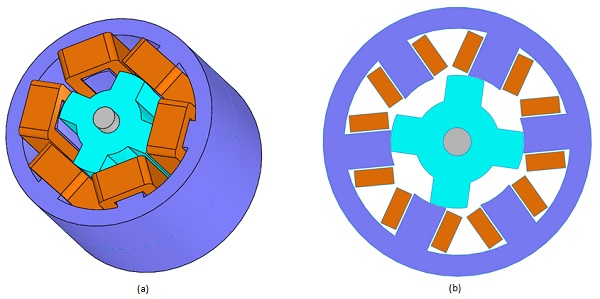Figure 2 - SRM, (a) 3D model, (b) 2D model

### Step 2: 2D FEM simulation

2D simulation of the SRM is performed next using MotorWizard.

Two windings belonging to the same phase aligned with the rotor pole are defined for this analysis. Each winding phase contains 20 turns and carries 4A DC current. Both the rotor and stator are made of non-oriented silicon steel M 27. The BH curve of M 27 is shown in Figure 3.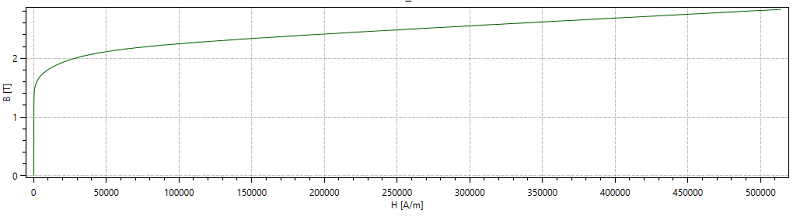Figure 3 - B-H curve of M 27

Figure 4 shows the generated mesh in the 2D model. It is possible to apply mesh refinement control in edges and surfaces. Here, a mesh control with smaller element size is applied on the air gap between the aligned poles. The air gap is modeled as a thin ring between the rotor and stator.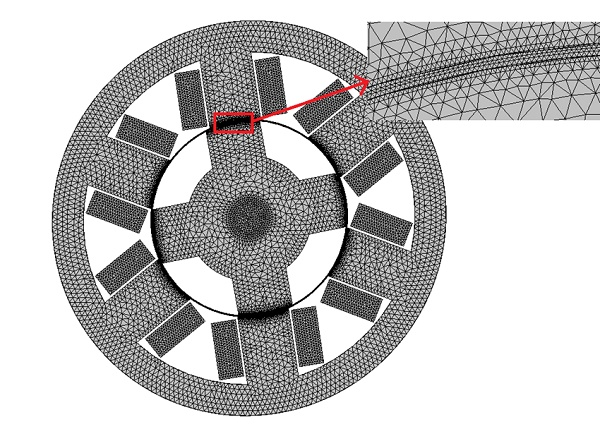Figure 4 - Meshed model

Magnetostatic module of MotorWizard computes and generates the following results: Magnetic flux density, magnetic field intensity, applied current density, Back EMF, inductance matrix, DC resistance, flux linkage, etc. Different shapes and types of plots are created. Since the static torque results will be evaluated versus rotor angle, a parametric analysis is defined.

## Results

Figure 5 contains the plot of magnetic flux density in the unaligned position (zero degree). The unaligned position is defined when the rotor pole is located across from the stator slot in such a way that the reluctance of the motor is at its maximum. From Figure 5, the peak value of the magnetic flux is about 0.16 T. Figure 6 shows the equipotential contours of the magnetic potential A which are equivalent to the magnetic flux lines (All current is assumed to flow perpendicular to the cross section). The plot demonstrates a discontinuity in the flux lines because of the high magnetic reluctance in this position (large air gap).

The magnetic flux density in the aligned position (45 degree) is plotted in the Figure 7. The aligned position is identified when the rotor pole is fully opposite the stator pole. In this case, the magnetic reluctance is at its minimum. The peak value of the magnetic flux density is about 0.87 T. The flux lines are plotted in Figure 8. They are following the ferromagnetic path from stator to rotor components without discontinuity (small air gap).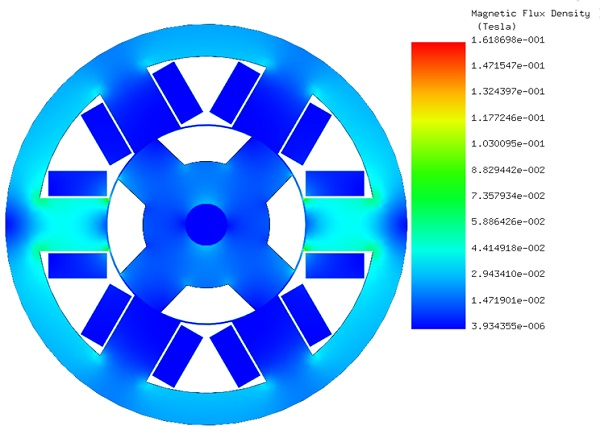Figure 5 - Magnetic flux density in the unaligned position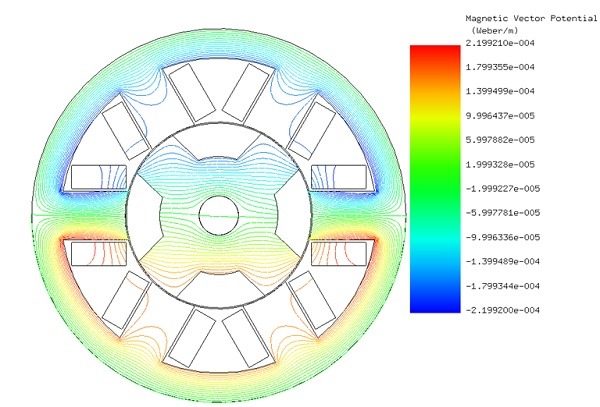Figure 6 - Magnetic potential equipotential contours in the unaligned position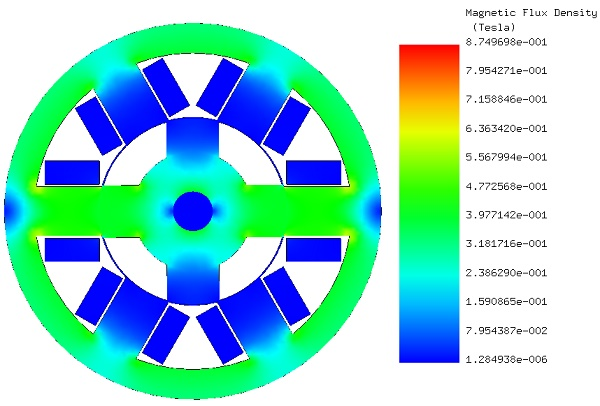Figure 7 - Magnetic flux density in the aligned position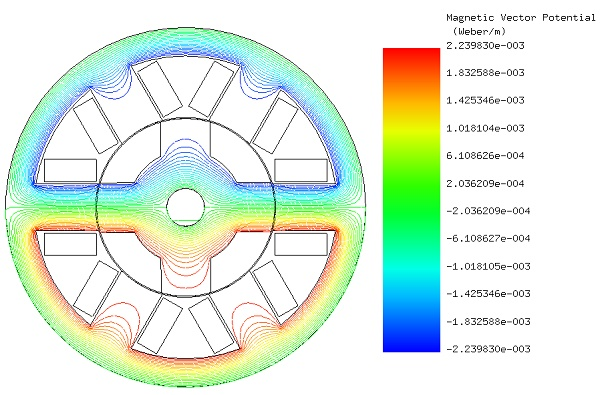Figure 8 - Magnetic potential equipotential contours in the aligned position

Figures 9 (a), 9 (b) and 9 (c) show the magnetic flux density respectively fringe plot, vector plot and lines plot at the rotor position of 16 deg. At this position, the magnetic flux and the torque reach their maximum values (0.913 T and 0.0304 Nm). The equipotential contours of A plot is attached to a cross section view of the motor 3D model in Figure 10. A comparison of the torque results is elaborated in Figure 11. The measurement data published in ref  show a maximum value of 0.0349 Nm at 16 deg, While the torque computed by MotorWizard and given by ref  have peak values of 0.03 and 0.029 respectively. This small gap can be justified by the end effect which is neglected in 2D simulation.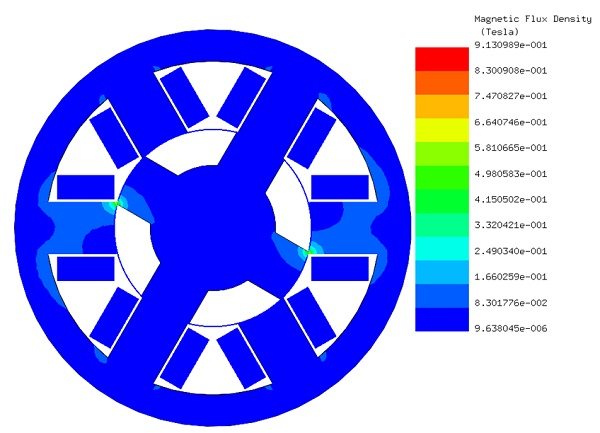Figure 9 (a) - Fringe plot of magnetic flux density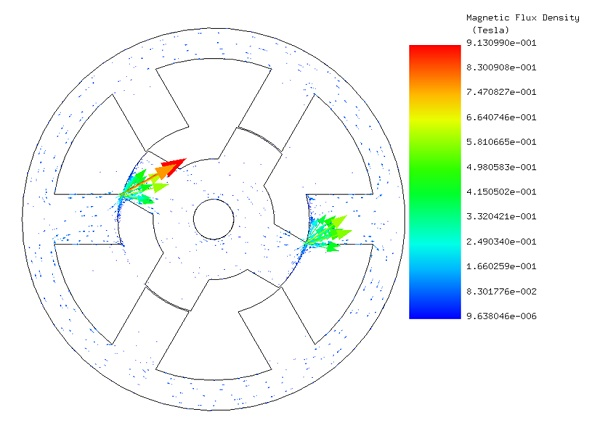Figure 9 (b) - Vector plot of the magnetic flux density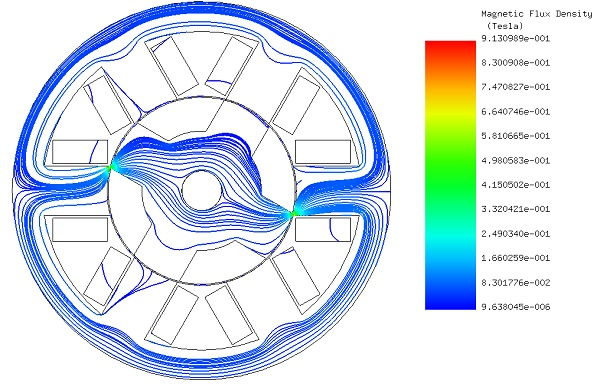Figure 9 (c) - Field lines plot of the magnetic flux density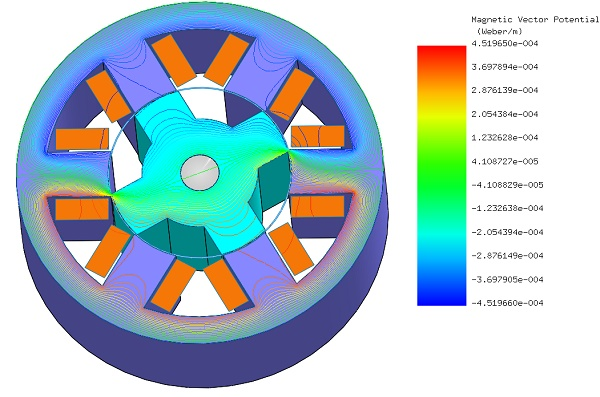Figure 10 - Equipotential contours of the magnetic potential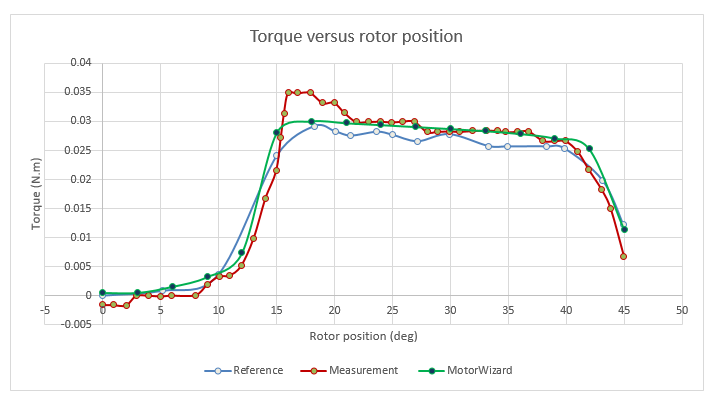Figure 11 - Static torque results

## Conclusion

A 2D model of a switched reluctance motor was created automatically and seamlessly and simulated using MotorWizard inside SOLIDWORKS. Magnetic flux and torque results were predicted and plotted versus the rotor angle. The results at the aligned and unaligned positions of the rotor and stator poles are highlighted and analyzed separately. Finally, the torque results computed with MotorWizard were compared to the measurement. This analysis can help to study the variation of the torque versus the rotor angle and identify the maximum and minimum torque and magnetic flux positions for the SR machine.

#### References

: Torsten Wichert, M.Sc. Design and Construction Modifications of Switched Reluctance Machines. Warsaw university of technology, Institute of Electrical Machines
: Kazuhiro Ohyama, Maged Naguib F. Nashed, Kenichi Aso, Hiroaki Fujii, and Hitoshi Uehara.Design Using Finite Element Analysis of Switched Reluctance Motor for Electric Vehicle. Department of Electrical Eng., Fukuoka Institute of Technology, Fukuoka, Japan,Electronics Research Institute, Cairo, Egypt and Meiwa Manufacturing Co., Ltd, Fukuoka, Japan. 0-7803-9521-2/06/\$20.00 §2006 IEEE.